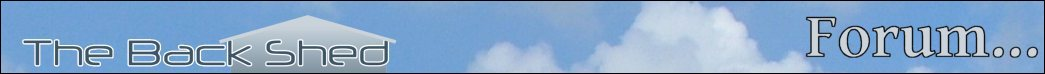Home JAQForum Ver 20.06 Log In or Join Active Topics Local Time 03:26 14 Apr 2021 Privacy Policy Jump to Select Sites home page Forum home page Windmills Solar EV's Electronics Microcontrollers PCB Manufacture Other Stuff Site News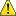Notice. New forum software under development. It's going to miss a few functions and look a bit ugly for a while, but I'm working on it full time now as the old forum was too unstable. Couple days, all good. If you notice any issues, please contact me.

Forum Index : Microcontroller and PC projects : BASIC10Liners - challenge for CMM2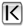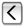Page 4 of 4
Author Message
vegipeteGuru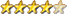Joined: 29/01/2013
Location: Canada
Posts: 721
 Posted: 01:14am 09 Mar 2021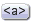Copy link to clipboard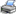Print this post

Got it!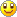Line 7 can be shortened by changing
If n<2Then:b=0:c=n*2-1:Else:b=n*2-5:c=0:EndIf
to
b=n*2-5:c=0:If n<2Then:b=0:c=n*2-1:EndIf

Volhout
GuruJoined: 05/03/2018
Location: Netherlands
Posts: 561
 Posted: 08:00am 09 Mar 2021Copy link to clipboardPrint this post

Hi Vegipete,

This is so challenging and exciting !!!

You can get it even shorter using booleans

From
b=n*2-5:c=0:If n<2Then:b=0:c=n*2-1:EndIf

To
c=(n<2)*(n*2-1):b=(n>1)*(n*2-5)

The 10 liner is the best thing that happened to me for a long time.
And you are the best.
- I will study the level decoder idea.
- drawing circles diameter 0 is what I thought of also, but I was afraid it would be visible (a single pixel).Wonder what it does to the speed. For every move you draw 169 circles (diameter 0).
- filling up the line ends with data.... that is smart. It compensates for the Loop and Next drawbacks.

Tonight I will give this version a try. I will postpone the data in the line ends, since I plan to look for more challenging levels, and put these in. I had some size 13 (that is where the 13 comes from) be haven't decoded them yet.

Thanks for the suggestions

@ TassyJim

I have made your suggested changes. The EXIT is a good find, the ":" after the then is not needed on the CMM1, but for compatibility to CMM2 I'll put it in.

Teamwork... Together we end up here...
s=13:Dim f(s,s),t(s,s):Do:Mode 4:z=9:Read x,y:For i=1To s:Read a\$:l=Val("&h"+a\$)
For j=1To s:f(i,j)=3And l:l=l\4:t(i,j)=(f(i,j)=1)
Next j,i:Print @(99,10)"TLS":f(x,y)=4:Do:d=0:If a\$="r"Then:Restore:Exit:EndIf
For i=1To s:For j=1To s:k=f(i,j):d=d+(k=1):o=120+z*(i-6):p=99+z*(j-6)
Line(o,p)-(o+z-2,p+z-2),3And k,BF:Circle(o+z/2-1,p+z/2-1),3*(k=4),6,,F:EndIf
Next j,i:If d+q=0Then:Exit:EndIf:Do:a\$=Inkey\$:Loop While a\$="":n=3And Asc(a\$)
c=(n<2)*(n*2-1):b=(n>1)*(n*2-5):e=x+b:g=y+c:If f(e,g)=3Then:b=0:c=0
ElseIf f(e,g)=2Then:If f(e+b,g+c)<2Then:f(e,g)=t(e,g):f(e+b,g+c)=2:Else:b=0:c=0
EndIf:EndIf:f(x,y)=t(x,y):x=x+b:y=y+c:f(x,y)=4:q=t(x,y)
Loop:Loop:Data 2,2,FFFF,D5F3,CBC3,C023,C3CF,EC0F,C0FF,FFFF,0,0,0,0,0

715 bytes (that is 9 lines of 80 characters. should change the title to NLS)

I'll test this version tonight....cross your thumbs.

Volhout
Edited 2021-03-09 18:36 by Volhout
If nothing goes right ... turn left

Volhout
GuruJoined: 05/03/2018
Location: Netherlands
Posts: 561
 Posted: 08:46pm 09 Mar 2021Copy link to clipboardPrint this post

This works.. Now trying to emulate "restore nn" to replay the current level.

s=13:Dim f(s,s),t(s,s):Do:Mode 4:z=9:Read x,y:For i=1To s:Read a\$:l=Val("&h"+a\$)
For j=1To s:f(i,j)=3And l:l=l\4:t(i,j)=(f(i,j)=1)
Next j,i:Print @(99,10)"TLS":f(x,y)=4:Do:d=0:If a\$="r"Then:Restore:Exit:EndIf
For i=1To s:For j=1To s:k=f(i,j):o=120+z*(i-6):p=99+z*(j-6)
Line(o,p)-(o+z-2,p+z-2),3And k,BF:Circle(o+z/2-1,p+z/2-1),3*(k=4),6,,F:d=d+(k=1)
Next j,i:If d+q=0Then:Exit:EndIf:Do:a\$=Inkey\$:Loop While a\$="":n=3And Asc(a\$)
c=(n<2)*(n*2-1):b=(n>1)*(n*2-5):e=x+b:g=y+c:If f(e,g)=3Then:b=0:c=0
ElseIf f(e,g)=2Then:If f(e+b,g+c)<2Then:f(e,g)=t(e,g):f(e+b,g+c)=2:Else:b=0:c=0
EndIf:EndIf:f(x,y)=t(x,y):x=x+b:y=y+c:f(x,y)=4:q=t(x,y)
Loop:Loop:Data 2,2,FFFF,D5F3,CBC3,C023,C3CF,EC0F,C0FF,FFFF,0,0,0,0,0

709 bytes
Edited 2021-03-10 06:53 by Volhout
If nothing goes right ... turn left

Volhout
GuruJoined: 05/03/2018
Location: Netherlands
Posts: 561
 Posted: 07:38am 10 Mar 2021Copy link to clipboardPrint this post

I managed to get 2 simple levels into the 10 lines, and when you press "r" you redo the current level.
For the 48 line contest, I may simply add 38 levels or so....

' TLS = ten line sokoban

' control by cursor keys-128 (128,129,130,131 => 0,1,2,3 => ^ V < >)
' playfield values 0=empty, 1=target, 2=block, 3=wall

'z   = size block
'x,y = position human
'e,g = new position human
'o,p = position onscreen
'n   = ascii value key
'b,c = direction human
'd   = done
'a\$  = key pressed
'l,m = vars for decoding rooms
'i,j = counters
'k   = value field (i,j)
'f() = playing field
't() = target locations for the boxes
's   = size playfield (sxs)
'q   = boolean (human on target)
'w   = level (0....nn)

'----------the compacted code---------

s=11:z=9:Dim f(s,s),t(s,s):Do:Mode 4:Read x,y:For i=1To s:Read v\$:l=Val("&h"+v\$)
For j=1To s:f(i,j)=3And l:l=l\4:t(i,j)=(f(i,j)=1):Next j,i:Print @(99,10)"TLS"
f(x,y)=4:Do:d=0:For i=1To s:For j=1To s:k=f(i,j):o=120+z*(i-6):p=99+z*(j-6)
Line(o,p)-(o+z-2,p+z-2),3And k,BF:Circle(o+z/2-1,p+z/2-1),3*(k=4),6,,F:d=d+(k=1)
Next j,i:If d+q=0Then:w=w+1:Exit:EndIf:Data 2,2,FFFF,D003,FF03,C003:Do:a\$=Inkey\$
Loop While a\$="":n=3And Asc(a\$):c=(n<2)*(n*2-1):b=(n>1)*(n*2-5):e=x+b:g=y+c
If f(e,g)=3Then:b=0:c=0:ElseIf f(e,g)=2Then:If f(e+b,g+c)<2Then:f(e,g)=t(e,g)
f(e+b,g+c)=2:Else:b=0:c=0:EndIf:EndIf:f(x,y)=t(x,y):x=x+b:y=y+c:f(x,y)=4:q=t(x,y)
If a\$="r"Then:Exit:EndIf:Data C083,C003,C003,FFFF,0,0,0,2,2,FFFF,D5F3,CBC3,C023
Loop:Restore:For i=1To(s+2)*w:Read j:Next i:Loop:Data C3CF,EC0F,C0FF,FFFF,0,0,0

'--------------extra data-------------
'simple 8x8
'Data 2,2,FFFF,D003,FF03,C003,C083,C003,C003,FFFF,0,0,0
'Data 2,2,FFFF,E103,FF03,C003,C003,C003,C003,FFFF,0,0,0
'8x8 field
Data 2,2,FFFF,D5F3,CBC3,C023,C3CF,EC0F,C0FF,FFFF,0,0,0
Data 5,2,FF0,C3F,FC03,C273,CE23,C44F,FF0C,3FC,0,0,0
Data 2,2,FFFF,C303,C0B3,DA13,CDEF,C10C,FFFC,0,0,0,0
'11x11 field
Data 2,3,FFC,3FC0C,30C8C,300BF,37F23,37203,3703F,37230,37F30,30030,3FFF0

If nothing goes right ... turn left

Volhout
GuruJoined: 05/03/2018
Location: Netherlands
Posts: 561
 Posted: 09:31pm 11 Mar 2021Copy link to clipboardPrint this post

This is going to be my entry to the challenge. I am finally happy with the performance, and the playability.

10 liner sokoban for CMM1
s=11:z=9:Dim f(s,s),t(s,s):Do:Mode 4:Read x,y:For i=1To s:Read a\$:l=Val("&h"+a\$)
For j=1To s:f(i,j)=3And l:l=l\4:t(i,j)=(f(i,j)=1):Next j,i:Cls:Print w,,"TLS"
f(x,y)=4:Do:d=0:For i=1To s:For j=1To s:k=f(i,j):o=120+z*(i-6):p=99+z*(j-6)
Line(o,p)-(o+z-2,p+z-2),3And k,BF:Circle(o+z/2-1,p+z/2-1),3*(k=4),6,,F:d=d+(k=1)
Next j,i:If d+q=0Then:w=w+1:Exit:EndIf:Data 9,2,FFC,3003,3003,30C3:Do:a\$=Inkey\$
Loop While a\$="":n=3And Asc(a\$):c=(n<2)*(n*2-1):b=(n>1)*(n*2-5):e=x+b:g=y+c
If f(e,g)=3Then:b=0:c=0:ElseIf f(e,g)=2Then:If f(e+b,g+c)<2Then:f(e,g)=t(e,g)
f(e+b,g+c)=2:Else:b=0:c=0:EndIf:EndIf:f(x,y)=t(x,y):x=x+b:y=y+c:f(x,y)=4:q=t(x,y)
If a\$="r"Then:Exit:EndIf:Data FC0,,FFC,3217,3203,3003,FFC,5,2,,,,FFFF,D5F3,CBC3
Loop:Restore:For i=1To(s+2)*w:Read a\$:Next i:Loop:Data C023,C3CF,EC0C,C0FC,FFC0

And 14 lines of extra levels
This makes up for a 80x24 editor screen from a terminal.
Data 8,5,,,,FC0,DC0,CFF,FE87,D83F,FFB0,370,3F0,6,3,,,3FF,3FF03,30FA3,30083,303FF
Data 3F300,C3F0,C570,FFF0,5,4,,,,FF0,C30,E3F,3DA3,3163,3163,3F03,3FF,7,7,,,,FF00
Data C3F0,C03F,CE07,C897,C87F,C3F0,FF00,6,6,,,3FF,3FC3,3C23,3CE3,3163,317F,32F0
Data 30C0,3FC0,9,7,,,,,FFFF,C017,C897,FABB,C897,C017,FFFF,7,6,,,FFFF,D503,D1B3
Data CB03,C23F,F30C,328C,3F0C,3FC,7,6,,,,3FF0,303F,3053,3373,32B3,3C23,C0F,FFC
Data 8,2,,,,FF0,C3F,FC03,C273,CE23,C44F,FF0C,3FC,6,2,,,,,FFFF,C303,C0B3,DA13
Data CDEF,C10C,FFFC,2,3,FFC,3FC0C,30C8C,300BF,37F23,37203,3703F,37230,37F30
Data 30030,3FFF0,6,3,,,,3FFFC,321DC,32FCF,31323,377C3,32043,31FFF,3FC00,10,7,,FF
Data 3FFCC3,300FE3,339003,331FEF,339C0C,3C1CEC,3F100C,30FFC,3FC00,5,10,,,3FFFC0
Data 3005C0,33F5FF,303003,32B0C3,3E3FCF,32000C,303F0C,3FF3FC,5,3,3FC0,30FF,F023
Data C2C3,CC8F,C0BC,FF0C,F0C,D0C,D5C,FFC,3,8,FFFFC,C3C0C,C008C,C0F0F,FC8C3,C803
Data FC3F,3570,3030,30F0,3FC0,9,7,,,,3FFF,3003,3333,3003,FF3F,C2A3,C153,FFFF
Data 10,10,3FF003,3333FF,303003,0,3FF3FF,8030,3FF3FF,0,3FF3FF,307333,FC303

How to play:
Sokoban is a game where a guy (yellow circle) has to shove boxes (green) to their destination (blue) within the warehouse walls (cyan). The guy can only push, not pull. He is controlled using the cursor keys. Once you make bad move, the "r" (lowercase only) let you replay the level.

The level is indicated in the top left corner. There are 2 levels in the 10 liner, 18 additional levels in the extra code.

Once you achieve a level, the next level is loaded. The aim is to finalize all 20 levels in one evening. A restart of the game forces you to start again with level 0.

However...you can cheat...
Variable "w" is the level that you go to after pressing "r". If you add this before the first line of the game, you can restart at any level by pressing "r" (example is level 16).
w=16

CMM2 owners should be able to run this by preceeding the program with
OPTION LEGACY ON

Happy Sokobanning...
Edited 2021-03-12 07:39 by Volhout
If nothing goes right ... turn left

Volhout
GuruJoined: 05/03/2018
Location: Netherlands
Posts: 561
 Posted: 09:57pm 11 Mar 2021Copy link to clipboardPrint this post

For the people that are exited enough to add levels to the game, you can simply add them between the last line and the one before.

What is important
Fields are maximum 11x11 determined by variable "s".
Larger are possible but all provided levels must be adapted.

The DATA statement needs 13 entries per level
1 - X coordinate guy (number 1 .. 11)
2 - Y coordinate guy (number 1 .. 11)
3.....13 : field information

The field information uses 2 bits per cel
&b00 = empty
&b01 = target (destination field)
&b10 = box
&b11 = wall

There is one caveat: due to the above definition it is not possible to add a level that starts out with one or more boxes already on it's destination.

If a field is smaller, simply add 0's until you have 13 entries.

Enjoy....
If nothing goes right ... turn leftPage 4 of 4Print this page

To reply to this topic, you need to log in.

© JAQ Software 2021